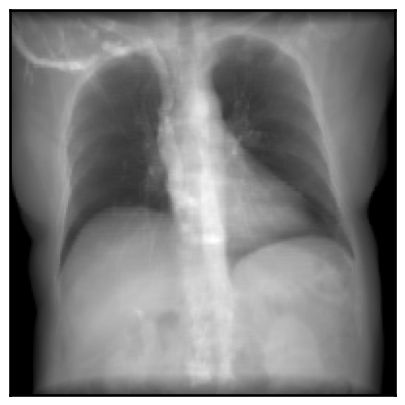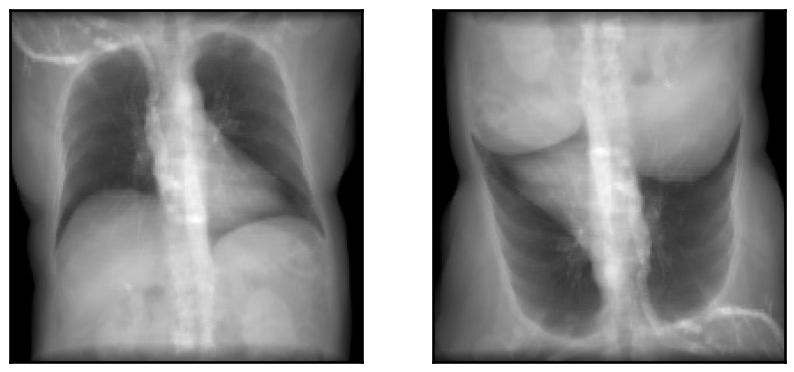# How to use `DiffDRR`

In-depth tutorial of the `DRR` module’s functionality
``````import matplotlib.pyplot as plt
import torch

from diffdrr.drr import DRR
from diffdrr.visualization import plot_drr``````

## DRR Generation

`DiffDRR` is implemented as a custom PyTorch module.

All raytracing operations have been formulated in a vectorized function, enabling use of PyTorch’s GPU support and autograd. This also means that DRR generation is available as a layer in deep learning frameworks.

Tip

Rotations can be parameterized with numerous conventions (not just Euler angles). See `diffdrr.DRR` for more details.

``````# Read in the volume and get the isocenter
bx, by, bz = torch.tensor(volume.shape) * torch.tensor(spacing) / 2

# Initialize the DRR module for generating synthetic X-rays
device = torch.device("cuda" if torch.cuda.is_available() else "cpu")
drr = DRR(
volume,  # The CT volume as a numpy array
spacing,  # Voxel dimensions of the CT
sdr=300.0,  # Source-to-detector radius (half of the source-to-detector distance)
height=200,  # Height of the DRR (if width is not seperately provided, the generated image is square)
delx=4.0,  # Pixel spacing (in mm)
).to(device)

# Set the camera pose with rotations (yaw, pitch, roll) and translations (x, y, z)
rotations = torch.tensor([[torch.pi, 0.0, torch.pi / 2]], device=device)
translations = torch.tensor([[bx, by, bz]], device=device)
img = drr(rotations, translations, parameterization="euler_angles", convention="ZYX")
plot_drr(img, ticks=False)
plt.show()``````We demonstrate the speed of `DiffDRR` by timing repeated DRR synthesis. Timing results are on a single NVIDIA RTX 2080 Ti GPU.

``33.8 ms ± 427 µs per loop (mean ± std. dev. of 7 runs, 10 loops each)``

### Sparse rendering

You can also render random sparse subsets of the pixels in a DRR.

Tip

Sparse DRR rendering can be useful in registration and reconstruction tasks when coupled with a pixel-wise loss, such as MSE.

``````# Make the DRR with 10% of the pixels
drr = DRR(
volume,
spacing,
sdr=300.0,
height=200,
delx=4.0,
p_subsample=0.1,  # Set the proportion of pixels that should be rendered
reshape=True,  # Map rendered pixels back to their location in true space,
# Useful for plotting, but can be disabled if using MSE as a loss function
).to(device)

# Make the DRR
img = drr(rotations, translations, parameterization="euler_angles", convention="ZYX")
plot_drr(img, ticks=False)
plt.show()````````8.56 ms ± 661 µs per loop (mean ± std. dev. of 7 runs, 100 loops each)``

## Batched DRR synthesis

The tensors for `rotations` are expected to be of the size `[batch_size, c]`, where `c` is the number of components needed to represent the rotation (`3, 4, 6, 10`). The tensors for `translations` are expected to be of the size `[batch_size, 3]`.

``````drr = DRR(
volume,
spacing,
sdr=300.0,
height=200,
delx=4.0,
).to(device)

rotations = torch.tensor(
[[torch.pi, 0.0, torch.pi / 2], [torch.pi, 0.0, -torch.pi / 2]], device=device
)
translations = torch.tensor([[bx, by, bz], [bx, by, bz]], device=device)
img = drr(rotations, translations, parameterization="euler_angles", convention="ZYX")
plot_drr(img, ticks=False)
plt.show()``````## Increasing DRR contrast

CT scans are easily segmented using Hounsfield units. We can use this to identify which voxels are air, soft tissue, or bone. By up or downweighting voxels corresponding to bones, we can change the contrast of generated DRRs.

``````# Completely downweight bones in CT (i.e., only soft tissue)
drr = DRR(
volume,
spacing,
sdr=300.0,
height=200,
delx=4.0,
).to(device)

rotations = torch.tensor([[torch.pi, 0.0, torch.pi / 2]], device=device)
translations = torch.tensor([[bx, by, bz]], device=device)

img = drr(
rotations,
translations,
parameterization="euler_angles",
convention="ZYX",
bone_attenuation_multiplier=0.0,
)
plot_drr(img, ticks=False)
plt.show()``````